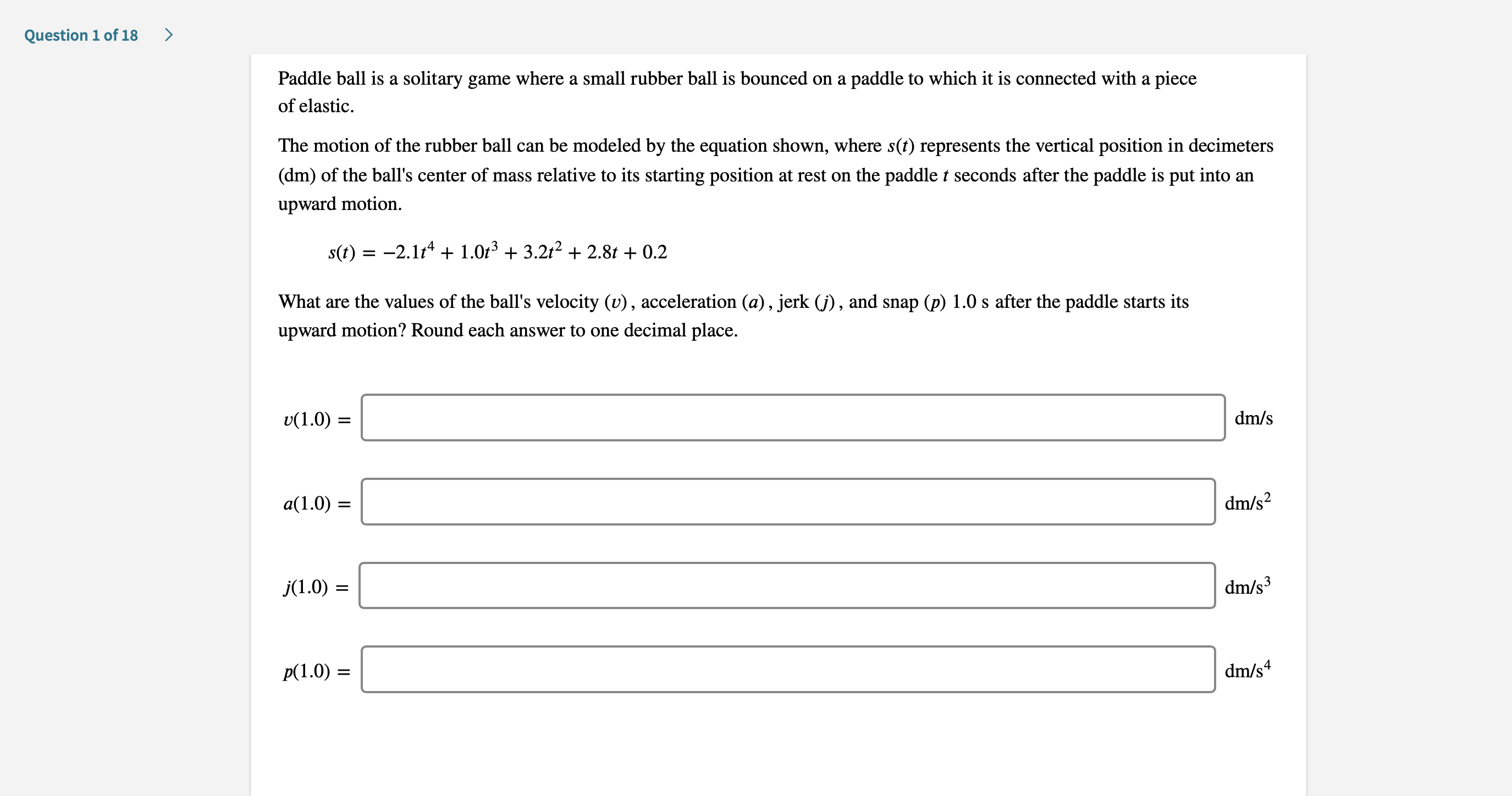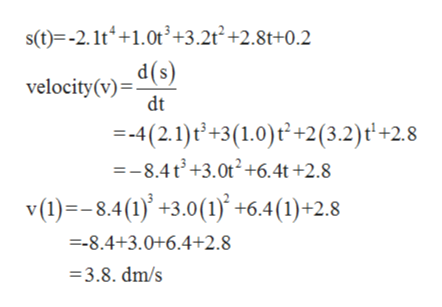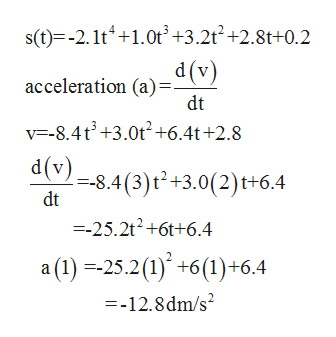# >Question 1 of 18Paddle ball is a solitary game where a small rubber ball is bounced on apaddle to which it is connected with a pieceof elasticThe motion of the rubber ball can be modeled by the equation shown, where s(t) represents the vertical position in decimeters(dm) of the ball's center of mass relative to its starting position at rest on the paddle t seconds after the paddle is put into anupward motions(t)2.14 1.0t3 3.2t2 2.8t 0.2+What are the values of the ball's velocity (v), acceleration (a), jerk (j), and snap (p) 1.0 s after the paddle starts itsupward motion? Round each answer to one decimal place.dm/sv(1.0)dm/s2a(1.0)dm/s3j(1.0)dm/s4Р(1.0) —

Question
3 viewshelp_outlineImage Transcriptionclose> Question 1 of 18 Paddle ball is a solitary game where a small rubber ball is bounced on a paddle to which it is connected with a piece of elastic The motion of the rubber ball can be modeled by the equation shown, where s(t) represents the vertical position in decimeters (dm) of the ball's center of mass relative to its starting position at rest on the paddle t seconds after the paddle is put into an upward motion s(t)2.14 1.0t3 3.2t2 2.8t 0.2 + What are the values of the ball's velocity (v), acceleration (a), jerk (j), and snap (p) 1.0 s after the paddle starts its upward motion? Round each answer to one decimal place. dm/s v(1.0) dm/s2 a(1.0) dm/s3 j(1.0) dm/s4 Р(1.0) — fullscreen
check_circle

Step 1

The motion of the rubber ball is modeled by the equation

S(t)= -2.1t4+1.0t3+3.2t2+2.8t+0.2

Find the values of velocity (v), acceleration (a), jerk(j) and snap(p) 1.0s after the paddle starts is upward motion?

Step 2

Given S(t)= -2.1t4+1.0t3+3.2t2+2.8t+0.2

Velocity (v)= ds/dthelp_outlineImage Transcriptioncloses(t) -2.1t+1.0t3+3.2t2+2.8t+0.2 velocity(v)- d(s) dt =-4(2.1)t+3(1.0)t+2(3.2)t'+2.8 =-8.4t+3.0t2+6.4t +2.8 v (1)-8.4(1)3.0(1) +6.4(1)+2.8 =-8.4+3.0+6.4+2.8 =3.8. dm/s fullscreen
Step 3

Acceleration(a)&nb...help_outlineImage Transcriptioncloses(t) -2. 1t+1.0t'+3.2t2+2.8t+0.2 (A)p dt acceleration (a) v-8.4t3+3.0+6.4t +2.8 dv) -8.4(3)t2+3.0(2)t+6.4 dt =-25.2t2+6t+6.4 a (1)25.2()+6(1)+6.4 =-12.8dm/s fullscreen

### Want to see the full answer?

See Solution

#### Want to see this answer and more?

Solutions are written by subject experts who are available 24/7. Questions are typically answered within 1 hour.*

See Solution
*Response times may vary by subject and question.
Tagged in

### Other